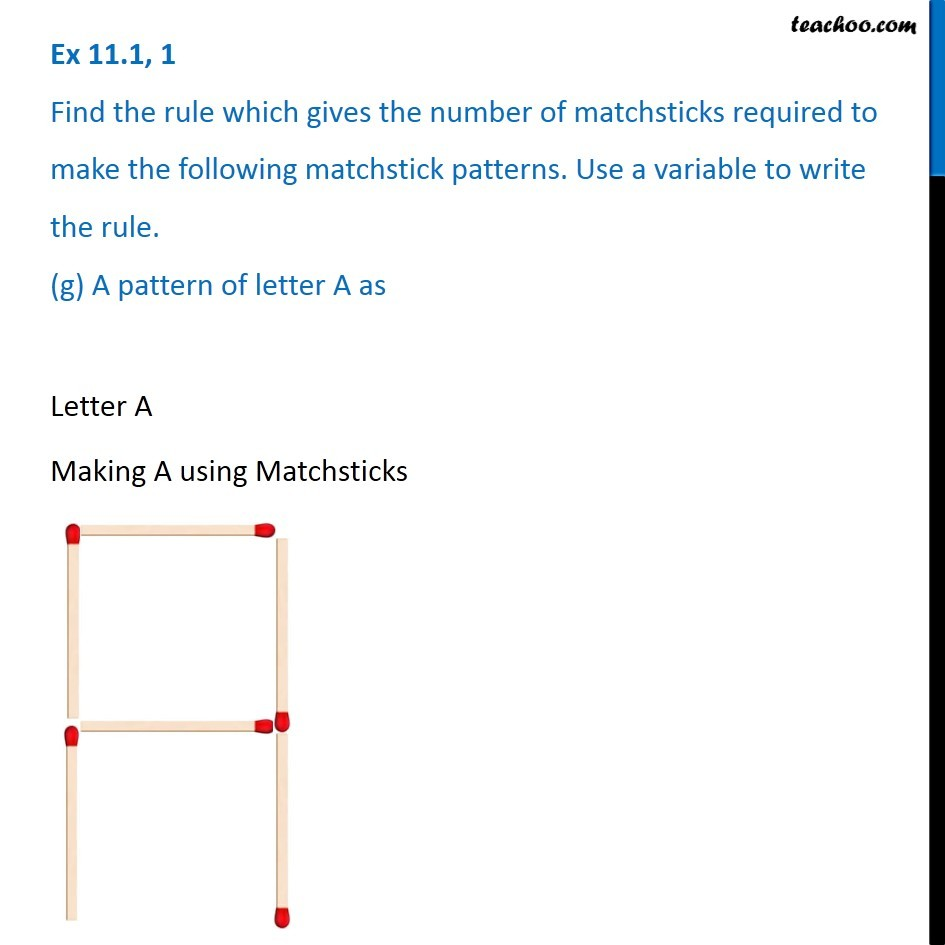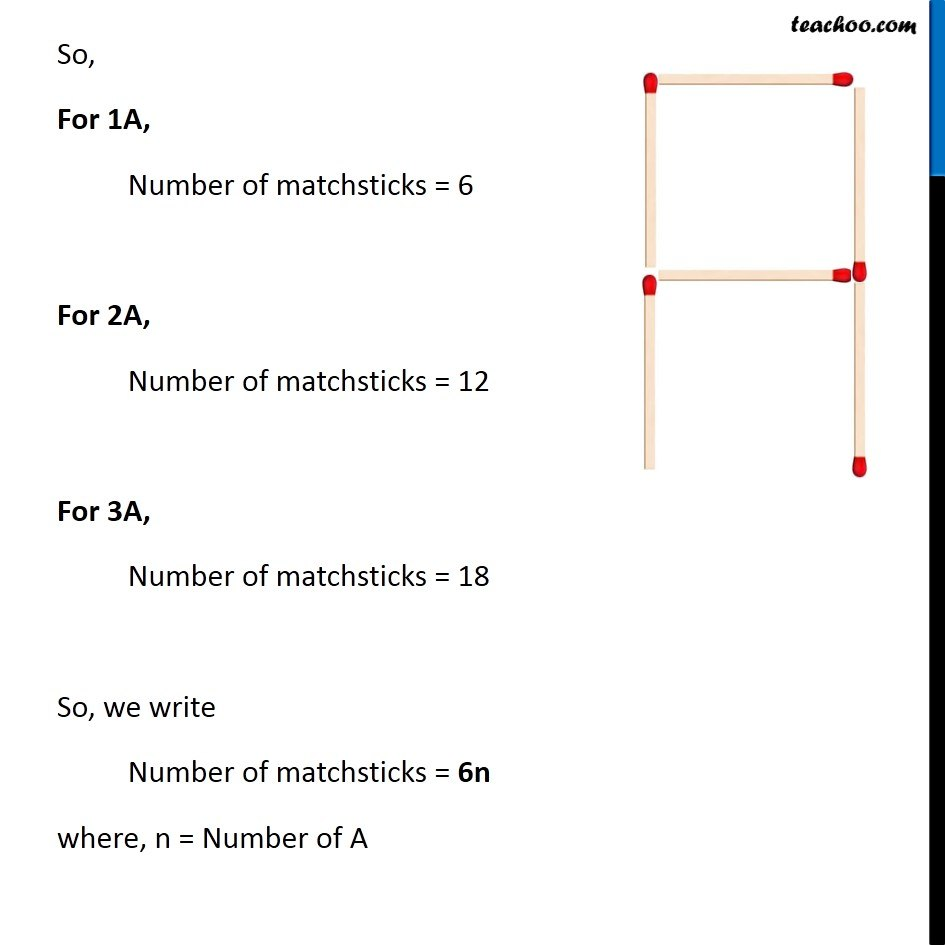Ex 11.1

Chapter 11 Class 6 Algebra
Serial order wiseLearn in your speed, with individual attention - Teachoo Maths 1-on-1 Class

### Transcript

Ex 11.1, 1 Find the rule which gives the number of matchsticks required to make the following matchstick patterns. Use a variable to write the rule. (g) A pattern of letter A as Letter A Making A using Matchsticks So, For 1A, Number of matchsticks = 6 For 2A, Number of matchsticks = 12 For 3A, Number of matchsticks = 18 So, we write Number of matchsticks = 6n where, n = Number of A